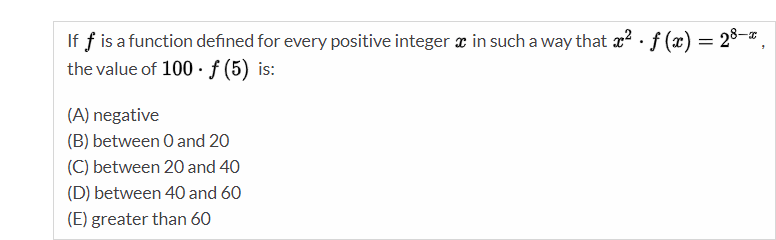GMAT Question of the Day - Daily to your Mailbox; hard ones only

 It is currently 06 Dec 2019, 10:52### GMAT Club Daily Prep

#### Thank you for using the timer - this advanced tool can estimate your performance and suggest more practice questions. We have subscribed you to Daily Prep Questions via email.

Customized
for You

we will pick new questions that match your level based on your Timer History

Track

every week, we’ll send you an estimated GMAT score based on your performance

Practice
Pays

we will pick new questions that match your level based on your Timer History

#### Not interested in getting valuable practice questions and articles delivered to your email? No problem, unsubscribe here.# If f is a function defined for every positive integer x in such a way

Author Message
TAGS:

### Hide Tags

GMATH TeacherP
Status: GMATH founder
Joined: 12 Oct 2010
Posts: 935
If f is a function defined for every positive integer x in such a way  [#permalink]

### Show Tags00:00

Difficulty:35% (medium)

Question Stats:73% (01:55) correct27% (01:35) wrongbased on 22 sessions

### HideShow timer Statistics

GMATH practice exercise (Quant Class 3)_________________
Fabio Skilnik :: GMATH method creator (Math for the GMAT)
Our high-level "quant" preparation starts here: https://gmath.net
InternB
Joined: 06 Feb 2018
Posts: 5
Location: Uzbekistan
Schools: Simon '21
GMAT 1: 650 Q47 V33GPA: 3.5
Re: If f is a function defined for every positive integer x in such a way  [#permalink]

### Show Tags

2
F(X)=(2^8-x)/x^2
F(5)=(2^3)/25=0.32
100*F(5)=32
GMATH TeacherP
Status: GMATH founder
Joined: 12 Oct 2010
Posts: 935
Re: If f is a function defined for every positive integer x in such a way  [#permalink]

### Show Tags

fskilnik wrote:

$${x^2} \cdot f\left( x \right) = {2^{8 - x}}\,\,\,\,\left( * \right)$$

$$? = 100 \cdot f\left( 5 \right)$$

$$x = 5\,\,\,\,\,\mathop \Rightarrow \limits^{\left( * \right)} \,\,\,\,\,25 \cdot f\left( 5 \right) = {2^3}\,\,\,\,\mathop \Rightarrow \limits^{ \cdot \,\,4} \,\,\,\,? = 4 \cdot {2^3} = 32$$

We follow the notations and rationale taught in the GMATH method.

Regards,
Fabio.
_________________
Fabio Skilnik :: GMATH method creator (Math for the GMAT)
Our high-level "quant" preparation starts here: https://gmath.netRe: If f is a function defined for every positive integer x in such a way   [#permalink] 17 Mar 2019, 17:36
Display posts from previous: Sort by

# If f is a function defined for every positive integer x in such a way